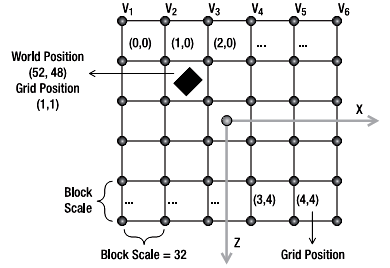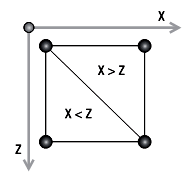# 10.5 查询地形高度// Get the position relative to the terrain grid
Vector2 positionInGrid = new Vector2( positionX - (StartPosition.X + Transformation.Translate.X), positionZ -
(StartPosition.Y + Transformation.Translate.Z));

// Calculate the grid position
Vector2 blockPosition = new Vector2( (int)(positionInGrid.X / blockScale), (int)(positionInGrid.Y / blockScale));private float GetHeight(float positionX, float positionZ)
{
float height = 	-999999.0f;
if (heightmap == null)
return height;
// Get the position relative to the terrain grid
Vector2 positionInGrid = new Vector2( positionX -(StartPosition.X + Transformation.Translate.X),
positionZ - (StartPosition.Y + Transformation.Translate.Z));

// Calculate the grid position Vector2
blockPosition = new Vector2( (int)(positionInGrid.X / blockScale), (int)(positionInGrid.Y / blockScale));

// Check if the object is inside the grid
if (blockPosition.X >= 0 && blockPosition.X < (vertexCountX - 1) &&
blockPosition.Y >= 0 && blockPosition.Y < (vertexCountZ - 1))
{
Vector2 blockOffset = new Vector2( blockPosition.X - (int)blockPosition.X,
blockPosition.Y - (int)blockPosition.Y);

// Get the height of the four vertices of the grid block
int vertexIndex = (int)blockPosition.X + (int)blockPosition.Y 	* vertexCountX;
float height1 = heightmap[vertexIndex + 1];
float height2 = heightmap[vertexIndex];
float height3 = heightmap[vertexIndex + vertexCountX +	1];
float height4 = heightmap[vertexIndex + vertexCountX];

// Top triangle float heightIncX, heightIncY;
if (blockOffset.X > blockOffset.Y)
{
heightIncX = height1 - height2;
heightIncY = height3 - height1;
}

// Bottom triangle
else
{
heightIncX = height3 - height4;
heightIncY = height4 - height2;
}

// Linear 	interpolation to find the height inside the triangle
float lerpHeight = height2 + heightIncX * blockOffset.X + heightIncY * blockOffset.Y;
height = lerpHeight * heightScale;
}
return height;
} 

2006 - 2023，推荐分辨率1024*768以上，推荐浏览器Chrome、Edge等现代浏览器，截止2021年12月5日的访问次数：1872万9823 站长邮箱